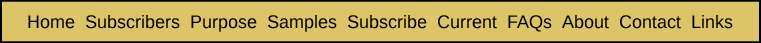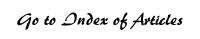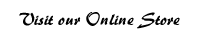Derivation of Formulas for the Probability of a Given ELS, Which Is Expected to Occur More Than Once, of Crossing a Specific Section of Text Appendix Five from Breakthrough: Encountering the Reality of the Bible Codes The goal of the last appendix was to provide formulas for the probability of a given ELS that occurs only once in a text of crossing a specific section of text. This probability is generally the same as the probability that the ELS with the smallest skip of all occurrences of the ELS in the entire text actually crosses the specific section of text — since in most cases there is only one ELS that has the smallest skip. It is of course possible to conceive of situations where there may be two or more ELSs that have the minimum skip. Since this is a rare situation, we will ignore this possibility for now. In this appendix, we will first use the probability derived in the last appendix to determine the probability that a given ELS that occurs more than once will cross a specific section of text. By the end of this appendix, we will proceed to use most of the formulas derived in previous appendices to start from the expected number of occurrences of an ELS and to determine the probability that it might cross a specific section of text. The expected number of occurrences will most likely not be a whole number but will rather be some mixed number like 0.36 or 2.37. This is because in reality, the number of occurrences might be zero or one or two or three or any of several larger numbers. And there are fractional probabilities that the actual number of occurrences will be any one of these specific numbers. The expected number of occurrences is a weighted average of all of the possible numbers of occurrences, with the weights being the probabilities themselves. To see this, let's go back to the example at the end of Appendix Two where the expected number of occurrences is 1.1 and the probability of any specific number of occurrences is given by the Poisson distribution. In our example, we were trying to estimate the probability that "gettysburg" would appear as an ELS within 12 entire books with an average length of 250 pages each. The Poisson distribution, with an expected number of occurrences of 1.1 (E), gave us these results:The expected number of occurrences can be obtained by taking the products of each of the possible number of occurrences times their probability and adding the sum of these products, as illustrated in the table below: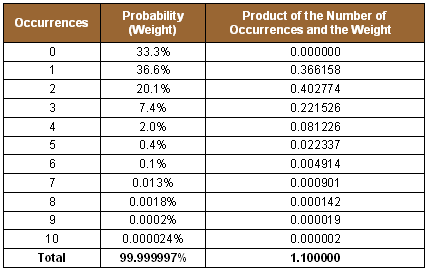Once we have determined the probabilities of any given number of occurrences of a particular ELS within a text, we will also need to derive a formula for the probability of one or more of these ELSs crossing the specific section of text. We will proceed by first deriving the formula for the probability that an ELS that occurs twice in the entire text of crossing the specific section of text. This formula will be based on the probability that an ELS that occurs only once in the entire text will cross. In this case, there are only two possible events: (1) the ELS crosses, and (2) the ELS does not cross. So the sum of the probabilities of both of these events must be 100%: P(Crosses) + P(Doesn't Cross) = 100%. Rearranging this, we get P(Doesn't Cross) = 100% – P(Crosses). Suppose, for example, that P(Crosses) = 10%. Then P(Doesn't Cross) is 100% - 10%, or 90%. Now P(Crosses) was derived in Appendix Four, so we can use the above formula to quickly derive P(Doesn't Cross). This is the probability that any single given ELS will fail to cross the specific section of text. As such, it is the same regardless of how many times the ELS actually occurs in the text. Now let us consider the case where the ELS occurs twice in the entire text. What is the probability that the first occurrence of the ELS will not cross the specific section of text? It is P(Doesn't Cross) = 100% – P(Crosses). What is the probability that the second occurrence of the ELS will not cross the specific section of text? It is, to a very small degree, dependent on whether or not the first occurrence of the ELS crossed the text. Let us take an example where there are 10 ELSs out of a possible 100 that could cross a specific section of text. Then we may summarize the possibilities in terms of the following table: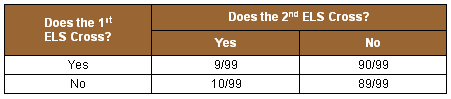In the above example, what is the probability that neither ELS crosses the text? It is (90/100) x (89/99) = 80.9090909%. This is very close to 90% x 90%, or 81.0%. Furthermore, when we are dealing with situations where the number of possible ELSs is very large, for all practical purposes we may assume that the event of the second ELS crossing (or not crossing) the text is independent of whether or not the first ELS crossed. Consider the example of where there are exactly 1 million possible ELSs and 100,000 of them could cross the text. Then the above table becomes: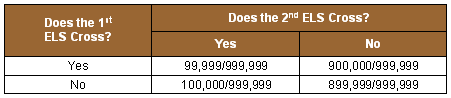In this case, the probability that neither the first nor the second ELS cross the specific section of text is (900,000/1,000,000) x (899,999/999,999), or 80.9999909%. This is extremely close to 81.0%. Because of this, we may assume, as a very close approximation, that the probability of not crossing is the same for the second occurrence of the ELS. So we again have P(Doesn't Cross) = 100% – P(Crosses). So, we may assume that for both the first and second occurrences of the ELS, the probability that the ELS will not cross will be 90%. We have seen that the probability the second ELS won't cross is virtually unaffected by whether or not the first ELS crossed the specific section. In other words, these two events are, for all practical purposes, independent of one another. Because of this virtual independence, the probability that neither ELS will cross will be the product of P(Doesn't Cross) for the first ELS and P(Doesn't Cross) for the second ELS. Therefore, the probability that neither will cross is (100% – P(Crosses)) * (100% – P(Crosses)) = (100% – P(Crosses)) ^ 2. In summary, then, in our example, the probability that neither ELS will cross is 90% * 90% = 81%. The above relationship can easily be generalized into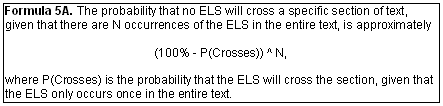The real concern about using Formula 5A is how close the approximation it provides is to the exact probability. What if we have a situation where there are 20 or 50 rows instead of just two? In the above example, where we start with a 90% probability of not crossing, if we use 90% to the tenth power as an approximation for (900,000/1,000,000) x (899,999/999,999) x (899,998/999,998) x . . . . . . x (899,993/999,993) x (899,992/999,992) x (899,991/999,991), then the approximation is only off by 0.0005%, since 34.8678440% is only that much more than the exact answer of 34.8676697%. Similarly, if there are 50 rows, then the approximation of 0.5153775% is only 0.0136125% more than the exact answer of 0.5153074%. Now that we have in Formula 5A a way of quickly deriving a very close approximation to the probability of no ELS crossing the specific section of text, we can proceed to find a way to use that approximation to estimate the probability that one or more ELSs cross the specific section of text. We will start by noting that all possible events may be grouped into one of two categories: (1) none of the ELSs cross the section, and (2) one or more ELSs cross the section. This gives us 100% = Probability(No Crossing ELSs) + Probability(One or More Crossing ELSs). From this we obtain the following: Probability (One or More Crossing ELSs) = 100% – Probability (No Crossing ELSs). This gives us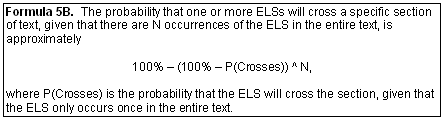In the case of our example, then, the probability that one or more ELSs will cross the given section of text is 100% – (90% ^ 2) = 100% – 81% = 19%. Let's take another example where E is 3.5 and the probability of the ELS crossing, given that the ELS occurs only once in the whole text is 5%. We will denote this probability by Probability(Crossing\One Occurrence) = 5%. In this notation the "\" should be read the same as the word "given." This is called a conditional probability. Our goal is to determine the probability that this ELS will cross the given section of text at least once. We will proceed by constructing the following table as the first phase of solving this problem.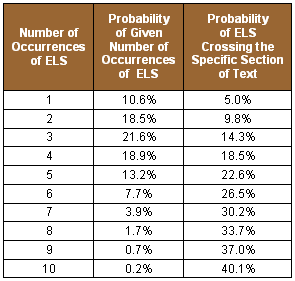This table breaks down the problem into ten different circumstances — according to the number of occurrences of the ELS in the entire text. We saw above how to obtain the probability of 9.8% for the case of two occurrences. It was derived by 100% – (95% ^ 2 ) = 100% – 90.25% = 9.75%. Similarly, for the case of three occurrences, the probability of crossing is 100% – (95% ^ 3) = 100% – 85.74% = 14.26%. Once we have completed the above table, we are then set up to determine the combined probability of a given ELS crossing a specific section of text — including the entire range of possible number of occurrences of the ELS in the full text. This can be calculated by filling out the table below. In that table, the first three columns are the same as those in the above table. The probabilities in the fourth column are obtained by taking the product of the probabilities in the second and third columns. For example, the probability that the given ELS will cross, given that there are three total occurrences in the entire text, is 3.1%. This was derived by multiplying the 21.6% chance that there will be exactly three occurrences of the ELS by the 14.3% probability of at least one ELS crossing the specific section of text.In the last column, we have cumulated the probabilities in the fourth column, starting from the top down. In this example, we have determined that there is a 16.0% probability that the given ELS will cross the specific section of text. The 16.0% is the sum of all of the individual probabilities for each possible number of occurrences of the ELS in the entire text. As noted previously, we can add on the additional constraint that the particular ELS that crosses the specific section of text will be the one that has the smallest skip of all occurrences of the ELS within the entire text. When we do that, we are restricting ourselves to the first row of the above table, because there is generally only one occurrence that has the minimum skip. Adding this type of restriction typically has a major impact on the probability of a chance occurrence. In the above example, it reduces the odds from 16.0% to 0.5%. As will be seen later in other examples, similar reductions in probability are normally the result of adding this constraint. In the above examples, we used the Poisson distribution to compute the probability of a given number of occurrences of an ELS within an entire text. In Appendix Two, we noted that the formula for the probability of any given number (k) of occurrences of an event, given that its expected number of occurrences is E, is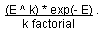We also noted that k factorial is the product of the number k itself times every whole number smaller than k. So 3 factorial is equal to 3 times 2 times 1, or 6. And 5 factorial is 5 times 4 times 3 times 2 times 1, or 120. When k becomes larger than 170, k factorial becomes such a huge number that most personal computers cannot handle it. For example, 170 factorial is computed by EXCEL to be 7.3 E +306, or 7.3 followed by 306 zeroes! You will get an error message if you try to compute the value of 171 factorial. There are many circumstances where the expected number of ELSs in a text will be greater than 170. Mathematicians typically handle such situations by using the normal distribution, or the bell shaped curve, as an approximation of the probabilities produced by the Poisson distribution. One characteristic of the Poisson distribution is that the standard deviation equals the mean, or expected value. If we also use this property for the normal distribution being used as an approximation for the Poisson distribution, we can produce tables like those in this chapter for situations where the expected number of occurrences is any number. For example, if E is 2,000, we will use a normal distribution with a mean of 2,000 and a standard deviation of 2,000 to estimate the probability of any given number of occurrences of the ELS. Since the normal distribution is a continuous distribution, however, when we want to compute the probability of exactly 2,000 occurrences of an ELS in a given text, we will compute this by taking the difference between the cumulative probabilities of the normal distribution at 1,999.5 and 2,000.5. Likewise, for ease of computation, when this distribution spans a wide range of numbers, we have approximated the probability of the given number of occurrences falling in a range from say 1,995 to 2,005 by taking the difference between the cumulative probabilities at these values and by calculating the other probabilities assuming the mid-point of this range (that is, 2,000).

 Enjoy finding your own Bible codes. Bible code search software is available in our online store. Subscribe Free! By signing up to be a member of The Isaac Newton Bible Research Society, you will have access to more than fifteen years of research by our team of Bible code researchers. Sign up to be a member today.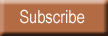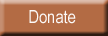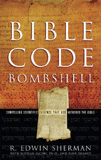Bombshell examines two massive, recently discovered clusters of codes in the Hebrew Old Testament. To read more about Bombshell, click here, or click below to order from Amazon today!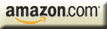| HOME | SUBSCRIBERS ONLY | OUR PURPOSE | FREE SAMPLES | CURRENT | | FAQs | ABOUT BIBLE CODES | SUBSCRIBE | CONTACT | Copyright © 2016 BibleCodeDigest.com